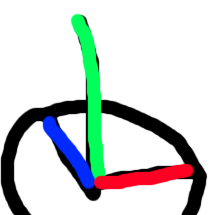# How to gain a random point infront of the user?

I’m trying to get a random point inside of a angle at the front of the user.
I want this point to be on a random range of a couple (1-10) studs on the Y and X axis infront of the user, with a variable Z axis.
Here’s an image of what I’m trying to achieve.With the y-axis being added into the equation, I’m trying to get the angle depicted as well as a random heighth.
How would this be done correctly?

Here’s a failed attempt of my shot at preforming this :

``````local randomCircle = math.random()  * math.pi
local Angle = CFrame.new(char.PrimaryPart.Position) * CFrame.Angles(0, angle + math.deg(90), 0) * CFrame.new(0, math.random(-3,3), -7)
--my equation got wrong and wronger
``````

This code when placed inside a LocalScript in StarterPlayer in StarterCharacterScripts

``````local Root = script.Parent:WaitForChild("HumanoidRootPart")

while true do

local Part = Instance.new("Part")
Part.Anchored = true
Part.Size = Vector3.new(1, 1, 1)
Part.Color = Color3.new(1, 0, 0)
Part.CanCollide = true
Part.Name = "PartX"
Part.Transparency = 0
Part.Position = Root.CFrame * Vector3.new(math.random(-10, 10), 5, math.random(-20, -10))
Part.Parent = game.Workspace

wait(1)
Part:Destroy()

end
``````
2 Likes